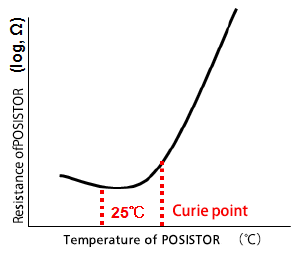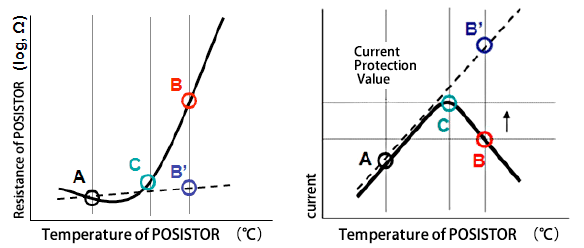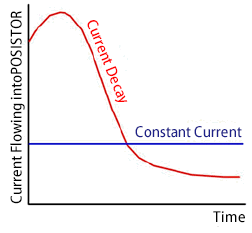# PTC Thermistors (POSISTOR) PTC Thermistors (POSISTOR)

Basic Knowledge of PTC Thermistors (POSISTOR)

- What is a PTC Thermistor?
Positive Temperature Coefficient Thermistor -

PTC Thermistors are an electronic component where the resistance remains almost constant near room temperatures. However, when the temperature exceeds a constant temperature, the resistance suddenly increases. "POSISTOR" is a registered trademark of Murata Manufacturing Co., Ltd.

## Feauture

The distinctive characteristics of "POSISTOR" can be acquired by adding a small amount rare earth elements into barium titanate (BaTiO3) .
Electrodes are formed in ceramics which use barium titanate as the main ingredient to create POSISTOR, and lead types and chip types are widely used.

The three characteristics of POSISTOR can be illustrated as in the following.

### Resistance - Temperature Characteristic

The resistance almost remains constant between room temperature (25°C) and the Curie point.
When the temperature exceeds the Curie point, the resistance suddenly increases. Utilizing this characteristic, abnormal conditions where a circuit is overheated beyond a specified temperature are detected, and the circuit can be shut down.What can be done by utilizing this characteristic?
When the temperature becomes more than the detection temperature, a POSISTOR can reduce the current flow!

Example, LED bulbs;
LED elements which is the core of LED bulbs, are electronic components that are extremely weak to heat.
When a large current flows through an LED element while heat is applied to the LED element, the LED element will be damaged.

The POSISTOR comes into play in such conditions! !

The POSISTOR detects the temperature around the LED element, and when the temperature reaches a specified temperature (detection temperature), the resistance of the POSISTOR suddenly increases to reduce the current flow. Accordingly, the POSISTOR prevents damage of LED elements by heat.

Since the resistance of a POSISTOR increases suddenly, the digital conversion of the temperature information is not required.
The temperature can be detected with a simple circuit!

Murata offers a variety of POSISTOR, from a lowCurie point of 40°C to as high as 130°C.

### Static Characteristic (Voltage-Current Characteristic)

The relationship between the current and voltage, when a voltage is applied to a POSISTOR is shown in the following figure.In the figure, the solid line shows the characteristics of a POSISTOR, and the dotted line shows the characteristics of the fixed resistance.
First, let's take a look at the relational figures of the resistance and temperature.

The fixed resistance shows an almost constant resistance, even though the temperature increases. (B' Point)
On the other hand, the resistance of a POSISTOR suddenly increases from the C point (Curie point) (B Point)

Next, let's take a look at the relationship between the current and voltage.

According to Ohm's Law, the current of the fixed resistance increases along with the application of a voltage.

On the other hand, the current in a POSISTOR remains the same as the fixed resistance up the C point, according to Ohm's Law.
However, when the current exceeds the C point due to self-heating, and the resistance of the POSISTOR itself increases, the current of the POSISTOR decreases along with the increase in the voltage.
Thus, the POSISTOR has a characteristic to maintain electric power at a constant.

What can be done by utilizing this characteristic?

• Heater
The POSISTOR is utilized in constant temperature heating elements, heaters and etc., taking advantage of these characteristics. The POSISTOR is different than a Nichrome heater, etc., and maintains a constant temperature without performing ON/OFF control.
• Overcurrent Protection
When an anbnormality occurs in an electronic circuit, a large current (overcurrent) will flow. Utilizing this characteristic, the POSISTOR limits the current in the circuit, so that an overcurrent does not flow into other electronic components, when this overcurrent flows. The POSISTOR limits the current in a circuit, to protect from overcurrents.

### Dynamic Characteristic (Current - Time Characteristic)

The following figure shows the relationship between the current and time when a voltage is applied to a POSISTOR. The red line shows the characteristic of the POSISTOR, and the blue line shows the characteristic of the fixed resistance.

As shown in the figure, a constant current flows in the fixed resistance, regardless of the elapsed time.

On the other hand, when a voltage is applied to a POSISTOR, the characteristic as shown in the figure is indicated. A large current flows because the resistance is low at the moment a voltage is applied, and the resistance increases by the self-heating of the POSISTOR along with the elapsed time, and the current which flows into the POSISTOR decreases.Many things can be realized using a POSISTOR! !

A POSISTOR allows for the initial inflow of a large current, and subsequently the current can be decreased by the self-heating.

For example, a compressor used in refrigerators.
The compressor is equipped with a motor, and a large current is required to start the motor. A POSISTOR is used because the components which allow the initial inflow of a large current, and reduces the current after a certain time has passed, is required!